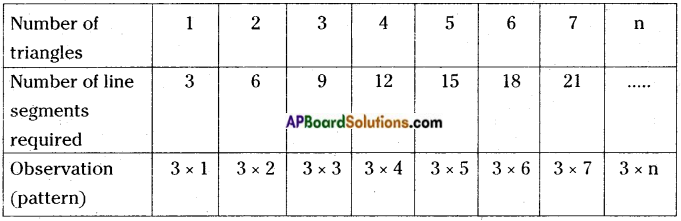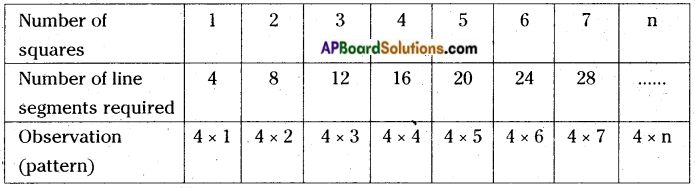# AP Board 6th Class Maths Notes Chapter 7 Introduction to Algebra

Students can go through AP Board 6th Class Maths Notes Chapter 7 Introduction to Algebra to understand and remember the concepts easily.

## AP State Board Syllabus 6th Class Maths Notes Chapter 7 Introduction to Algebra

→ Expressions: 7 + 14 – 6 & 12 – 8 + 56 +…. such mathematical sentences are called numerical expressions.

When we try to make some general patterns we need to involve some constants and variables in the statements.
Eg: If three line segments are needed to construct a triangle, then the number of line segments needed to construct n-number of triangles is 3 × n.Here the number of line segments required for a given number of triangles = 3x The value of the numeral is fixed and called a constant and x can take any value from 1, 2, 3, 4, 5, 6,…So we say that x value is not fixed and varies and hence x is a variable.
If four line segments are needed to construct a square, then the number of line segments needed to construct n-number of squares is 4 × n.Here the number of line segments required for a given number of triangles = 4x The value of the numeral 4 is fixed and called a constant and x can take any value from 1, 2, 3, 4, 5, 6, ….

So we say that x value is not fixed and varies and hence x is a variable.

→ A variable is an alphabet used to stand for a number.
A variable can like any value; it has no fixed value, but it is a number. We can perform binary operation such as addition, subtraction, multiplication and divisions on them.
Eg: If x is a variable,
then 5 more than x is x + 5
5 less than x is x – 5
5 times x is 5x
One fifth of x is $$\frac{1}{5}$$.→ A variable allows us to express relations in any special situation. Variables allow us to express many common rules of Geometry and Arithmetic in a more general way.
Eg: If the side of a square is s,
then its Area A = s × s
Perimeter = 4 × s
If the length and breadth of a rectangle are l & b, then its
Area A = l × b
Perimeter is P = 2 × (l + b).
The general form of an odd number = 2 × n + 1 = 2n + 1;
Even number = 2 × n = 2n
In the above we have number of line segments required for a given number of triangles is 3 × n.
Suppose the number of triangles is 4, then 4n = 12, this is an equation.
An expression involving the equality (=) symbol is called an equation.
The part l value of the expression on the left of the equality (=), is called LEFT HAND SIDE or L.H.S.
The part l value of the expression on the right of the equality (=), is called RIGHT HAND SIDE or R.H.S.
If the L.H.S. is not equal to R.H.S., then we do not get an equation.
Eg : 8 + 13 ≠ 15
2 + 3 < 9 – 2
56 + 3 > 25 + 5→ Solution or root of an equation:
Solution of an equation is the value of the variable for which L.H.S and R.H.S are equal. The solution is also called the root of the equation.
Eg : Solution of x – 8 = 4 is x = 12
For the equation x + 3 = 8, x = 7 is not a solution.
We find the solution of an equation by Trial & Error method.
Trial -error method is a process in which the solution of an equation is found by taking some arbitrary values for the variable.